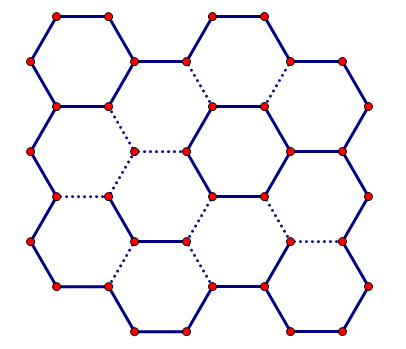시간 제한 메모리 제한 제출 정답 맞은 사람 정답 비율
6 초 512 MB 2 2 2 100.000%

## 문제

A honeycomb is a mass of hexagonal prismatic cells, where each cell has six adjacent cells, and every two adjacent cells share an edge. In addition, some edges are traversable but others are not.

Hamilton is an industrious worker bee. Every day he works for connecting or disconnecting some pairs of adjacent cells in a honeycomb. In a few days, he will have a great chance to design a tiny block of cells by himself. This block is disconnected from its outside and it could be represented as cells in n rows and m columns, like the figure shown below.To cut off the connection of two cells, he may have to change some edges into untraversable. He is wondering the minimum number of edges he has to change so that two specified cells in the block would be disconnected. Could you please help him find out the minimum number of changed edges for every two special cells in this block? To avoid huge output data, you are asked to report the sum of these minimum numbers of edges.

## 입력

The input contains several test cases. The first line contains an integer T indicating the number of test cases. The following describes all test cases. For each test case:

The first line contains two integers n and m.

The following (4n+ 3) lines describe the block, where each line contains at most (6m+ 3) characters. Odd lines contain grid vertices represented as plus signs (“+”) and horizontal edges, while even lines contain diagonal edges.

Specifically, a cell is described as 6 vertices and 6 edges. All edge characters will be placed exactly between the corresponding vertices, such that edges are described as following:

• Its upper boundary or lower boundary is represented as three consecutive minus signs (“-”) if the edge is untraversable, or three consecutive spaces (“ ”) otherwise;
• Each one of its diagonal edges is represented as a single space if the edge is traversable, or otherwise as a single forward slash (“/”) or a single backslash (“\”) character, depending on the direction of the edge.

Besides, there is an asterisk (“*”) character at the center of each special cell. All other characters in the input will be spaces, and no input line will contain trailing spaces.

## 출력

For each test case, output a line containing “Case #x: y” (without quotes), where x is the test case number starting from 1, and y is the answer to this test case.

## 제한

• 1 ≤ T ≤ 100
• 2 ≤ n, m ≤ 100
• It is guaranteed that every non-special cell has no traversable edge.
• The sum of the numbers of special cells in all test cases does not exceed 3000.

## 예제 입력 1

2
2 2
+---+
/     \
+   *   +---+
\     /     \
+---+   *   +
/           /
+   *   +   +
\           \
+---+   *   +
\     /
+---+
2 3
+---+       +---+
/     \     /     \
+   *   +---+       +
\           \     /
+---+   *   +---+
/                 \
+   *   +---+   *   +
\                 /
+---+   *   +---+
\     /
+---+


## 예제 출력 1

Case #1: 6
Case #2: 16